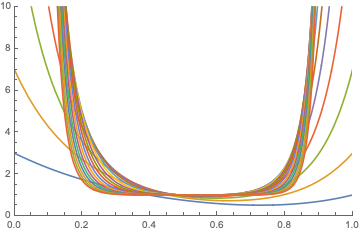# What is the largest possible probability that a random matrix over $\mathbb{F}_2$ is non-singular?

Suppose $$A(p, n)=(a_{ij}(p))_{i, j \leq n}$$ is an $$n\times n$$ random matrix over $$\mathbb{F_2}$$, with all its entries being i.i.d. and such that $$P(a_{ij}(p) = 1) = p$$, where $$p$$ is some real number from $$[0; 1]$$. What is the largest possible probability, that $$A(p, n)$$ is non-singular and with what $$p$$ is it reached?

Note, that $$A(p, n)$$ is non-singular iff $$\det(A(p, n)) = 1$$.

Solution for $$n=1$$:

$$\det(A(p, 1)) = 1$$ with probability $$p$$. The maximum of $$\det(A(p, 1))$$ is $$1$$ and it is reached with $$p = 1$$.

Solution for $$n = 2$$:

$$\det(A(p, 2)) = 1$$ with probability $$2p^2(1 - p^2)$$. The maximum of $$P(\det(A(p, 2))=1)$$ is $$\frac{1}{2}$$ and it is reached with $$p = \frac{1}{\sqrt{2}}$$.

However, I would like to know some sort of general formula (or at least asymptotics).

After I failed to solve this problem using determinants, I tried to prove this using the fact that a square matrix is non-singular iff its rows are linearly dependent. As there exists only one non-zero element in $$\mathbb{F_2}$$, we can write linear dependence of the vector system $$\{v_i\}_{i \leq n}$$ in $$\mathbb{F}_2^n$$ as $$\forall S \subset \{1, ... , n\}$$ such that $$S \neq \emptyset$$ we have $$\sum_{i \in S} v_i \neq \overline{0}$$. I know the probability that a given set of vectors with i.i.d. random entries Bernoulli distributed with parameter $$p$$ $$\{v_i\}_{i \leq k}$$ over $$\mathbb{F}_2^n$$ satisfy $$\sum_{i = 1}^k v_i \neq \overline{0}$$ is $$(1 - \frac{p((1 - 2p)^k - 1)}{1 - 2p})$$. However, I do not know how to proceed further in this direction.

This question on MSE

For $$n\rightarrow\infty$$ the probability $${\cal P}_\infty$$ that $$A(p,n)$$ is nonsingular becomes independent of $$p\in(0,1)$$, given by $${\cal P}_\infty=\prod_{i=1}^\infty(1-2^{-i})=0.2887880951$$ See theorem 3.2 in Properties of random matrices and applications (2007).
For finite $$n$$, there is a result that could be instructive, which is the expectation value $$E(p,n)$$ of the number of linear dependencies among the rows of $$A(p,n)$$. This is given by theorem 4.5, $$E(p,n)=2^{-n}\sum _{j=1}^n \binom{n}{j} \left(1+(1-2 p)^j\right)^n.$$ A plot of $$E(p,n)$$ as a function of $$p$$ becomes flatter and flatter with increasing $$n$$ (see below for $$n$$ up to 20), consistent with the understanding that for large $$n$$ the probability that the matrix is singular no longer depends on $$p$$.